# GMAT Math : DSQ: Calculating ratio and proportion

## Example Questions

### Example Question #1 : Ratio & Proportions

At Branchwood Middle School, there are 4 sixth graders for every 5 seventh graders and 6 seventh graders for every 5 eighth graders.  How many sixth graders are there?

2. There are 75 eighth graders at the middle school.

EACH statement ALONE is sufficient.

Statement (1) ALONE is sufficient, but statement (2) alone is not sufficient.

Statement (1) and (2) TOGETHER are NOT sufficient to answer the question asked, and additional data are needed.

BOTH statements TOGETHER are sufficient, but NEITHER statement ALONE is sufficient.

Statement (2) ALONE is sufficient, but statement (1) alone is not sufficient.

Statement (2) ALONE is sufficient, but statement (1) alone is not sufficient.

Explanation:

Statement 1: This information can already be determined from the original information.

Statement 2: The two ratios can be connected by the common element of seventh graders. Convert the two ratios so that the seventh grade value is the same in both. The ratio of sixth graders: seventh graders:eighth graders = 24:30:25.

Therefore,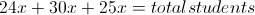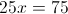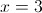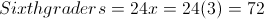### Example Question #1 : Ratio & Proportions

Fred is looking at a map and is wondering how far it is from Washington City to Bush Corner. He sees that on the map, they are three and a half inches apart. From the map distance between the two, how far is it in actual miles?

Statement 1: The distance from Adamsville to Clinton Ridge on the map is four and a half inches.

Statement 2: In actuality, it is 85 miles from Adamsville to Clinton Ridge.

BOTH statements TOGETHER are insufficient to answer the question.

BOTH statements TOGETHER are sufficient to answer the question, but NEITHER statement ALONE is sufficient to answer the question.

Statement 1 ALONE is sufficient to answer the question, but Statement 2 ALONE is NOT sufficient to answer the question.

EITHER statement ALONE is sufficient to answer the question.

Statement 2 ALONE is sufficient to answer the question, but Statement 1 ALONE is NOT sufficient to answer the question.

BOTH statements TOGETHER are sufficient to answer the question, but NEITHER statement ALONE is sufficient to answer the question.

Explanation:

Fred needs to know the ratio of actual miles to map inches to solve this problem; once he knows this, he can multiply this ratio by the map distance from Washington City to Bush Corner. Neither of the facts alone about the other two cities are helpful, but if he knows both, he can determine the ratio he needs to achieve his goal.

### Example Question #3 : Dsq: Calculating Ratio And Proportion

Last year, a computer shop had an average of 75 computers in stock at the start of each day, and it sold an average of 300 computers each week.

This year, the shop is expecting to sell an average of 732 computers each week.  The computer store wants to use last year's ratio of computers in stock to computers sold to decide how many computers to have in stock each day. What should this target number be?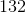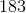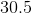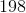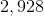Explanation:

We can set up a proportion to solve for the number of computers the store should keep in stock each day: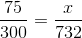Cross-multiply: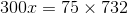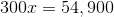Divide both sides by 300: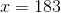The store should aim to have 183 computers in stock at the start of each day.

### Example Question #4 : Dsq: Calculating Ratio And Proportion

Eleanor has a a scale model of the Voyager I probe. What is the radius of the dish in the model?

I) The length of the antennae on the model is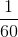the length of the actual antennae.

II) The radius of the dish on the actual probe isfeet.

Statement II is sufficient to answer the question, but statement I is not sufficient to answer the question.

Statement I is sufficient to answer the question, but statement II is not sufficient to answer the question.

Either statement is sufficient to answer the question.

Both statements are needed to answer the question.

Both statements are needed to answer the question.

Explanation:

The secret to this problem is to set up a proportion.

We are given the ratio of one part of the model from Statement I, and asked to find the length of another part.

We need both I) and II) to set up the following proportion.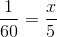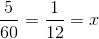So both statements are needed.

### Example Question #5 : Dsq: Calculating Ratio And Proportion

In a car dealership there areSUVs to everysedans, andsedans to everysports car. How many sedans are there?

1. There areSUVs.
2. There aresport cars.

Statement 1 and 2 are not sufficient, and additional data is needed to answer the question.

Both statements taken together are sufficient to answer the question, but neither statement alone is sufficient.

Statement 2 alone is sufficient, but statement 1 alone is not sufficient to answer the question.

Statement 1 alone is sufficient, but statement 2 alone is not sufficient to answer the question.

Each statement alone is sufficient.

Each statement alone is sufficient.

Explanation:

We can combine the given ratios into one ratio by using the least common mulitiple of 8 and 6, which is 24.

In order to arrive to 24, we need to multiply the first ratio by 3 and the second ratio by 4. We can then arrive to a single ratio of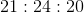Using our ratio, we have an equation: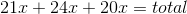Statement 1: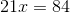then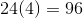sedans

so Statement 1 is sufficient to answer the question

Statement 2: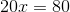thensedans

so Statement 2 is also sufficient to answer the question